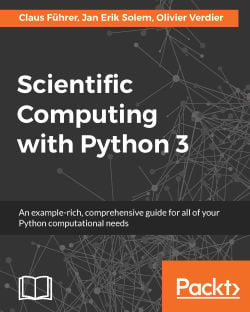### Scientific Computing with Python 34.7 (3 reviews total)
By Claus Führer , Jan Erik Solem , Olivier Verdier
• 7-day free trial Subscribe Access now
• \$39.99 eBook

• Constantly updated with 100+ new titles each month
• Breadth and depth in over 1,000+ technologies

Python can be used for more than just general-purpose programming. It is a free, open source language and environment that has tremendous potential for use within the domain of scientific computing. This book presents Python in tight connection with mathematical applications and demonstrates how to use various concepts in Python for computing purposes, including examples with the latest version of Python 3. Python is an effective tool to use when coupling scientific computing and mathematics and this book will teach you how to use it for linear algebra, arrays, plotting, iterating, functions, polynomials, and much more.

Publication date:
December 2016
Publisher
Packt
Pages
332
ISBN
9781786463517

## Chapter 1. Getting Started

In this chapter, we will give a brief overview of the principal syntactical elements of Python. Readers who have just started learning programming are guided through the book in this chapter. Every topic is presented here in a how-to way and will be explained later in the book in a deeper conceptual manner and will also be enriched with many applications and extensions.

Readers who are already familiar with another programming language will come across, in this chapter, the Python way of doing classical language constructs. It offers them a quick start to Python programming.

Both types of readers are encouraged to take this chapter as a brief guideline when zigzagging through the book. However, before we start we have to make sure that everything is in place and you have the correct version of Python installed together with the main modules for Scientific Computing and tools, such as a good editor and a shell, which helps in code developing and testing.

Read the following section, even if you already have access to a computer with Python installed. You might want to adjust things to have a working environment conforming to the presentation in this book.

## Installation and configuration instructions

Before diving into the subject of the book you should have all the relevant tools installed on your computer. We will give you some advice and recommend tools that you might want to use. We only describe public domain and free tools.

### Installation

There are currently two major versions of Python; the 2.x branch and the new 3.x branch. There are language incompatibilities between these branches and one has to be aware of which one to use. This book is based on the 3.x branch, considering the language up to release 3.5.

For this book you need to install the following:

• The interpreter: Python 3.5 (or later)
• The modules for scientific computing: SciPy with NumPy
• The module for graphical representation of mathematical results: matplotlib
• The shell: IPython
• A Python related editor: Spyder (refer to the following Figure 1.1, Spyder), Geany

The installation of these is eased by the so-called distribution packages. We recommend that you use Anaconda. The default screen of Spyder consists of an editor window on left, a console window in the lower right corner which gives access to an IPython shell and a help window in the upper right corner as shown in the following figure: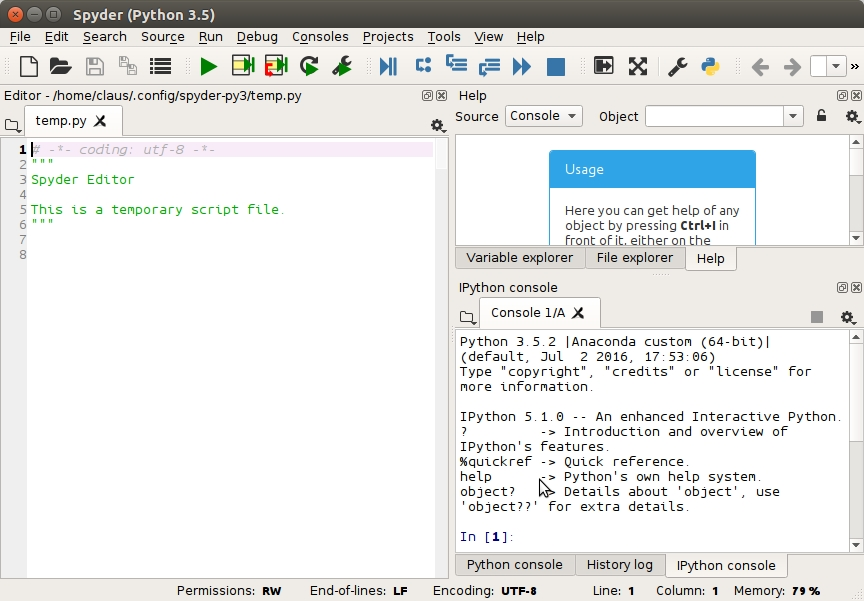Figure 1.1: The default screen of Spyder consists of an editor window on left, a console window in the lower right corner which gives access to an IPython shell and a help window in the upper right corner.

### Anaconda

Even if you have Python pre-installed on your computer, we recommend that you create your personal Python environment that allows you to work without the risk of accidentally affecting the software on which your computer's functionality might depend. With a virtual environment, such as Anaconda, you are free to change language versions and install packages without the unintended side-effects.

If the worst happens and you screw things up totally, just delete the Anaconda directory and start again. Running the Anaconda installer will install Python, a Python development environment and editor (Spyder), the shell IPython, and the most important packages for numerical computations, for example SciPy, NumPy, and matplotlib.

You can install additional packages with `conda install` within your virtual environment created by Anaconda (refer for official documentation from).

### Configuration

Most Python codes will be collected in files. We recommend that you use the following header in all your Python files:

```from scipy import *
from matplotlib.pyplot import *```

With this, you make sure that all standard modules and functions used in this book, such as SciPy, are imported. Without this step, most of the examples in the book would raise errors. Many editors, such as Spyder, provide the possibility to create a template for your files. Look for this feature and put the preceding header into a template.

### Python Shell

The Python shell is good but not optimal for interactive scripting. We therefore recommend using IPython instead (refer to  for the official documentation). IPython can be started in different ways:

• In a terminal shell by running the following command:`ipython`
• By directly clicking on an icon called Jupyter QT Console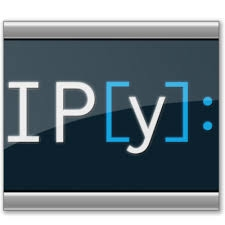• When working with Spyder you should use an IPython console (refer to Figure 1.1, Spyder).

### Executing scripts

You often want to execute the contents of a file. Depending on the location of the file on your computer, it is necessary to navigate to the correct location before executing the contents of a file.

• Use the command `cd` in IPython in order to move to the directory where your file is located.
• To execute the contents of a file named `myfile.py`, just run the following command in the IPython shell
```      run myfile
```

### Getting Help

Here are some tips on how to use IPython:

• To get help on an object, just type `?` after the object's name and then `return`.
• Use the arrow keys to reuse the last executed commands.
• You may use the Tab key for completion (that is, you write the first letter of a variable or method and IPython shows you a menu with all the possible completions).
• Use Ctrl+D to quit.
• Use IPython's magic functions. You can find a list and explanations by applying  `%magic`  on the command prompt.

### Tip

You can find out more about IPython in its online documentation, .

### Jupyter – Python notebook

The Jupyter notebook is a fantastic tool for demonstrating your work. Students might want to use it to make and document homework and exercises and teachers can prepare lectures with it, even slides and web pages.

If you have installed Python via Anaconda, you already have everything for Jupyter in place. You can invoke the notebook by running the following command in the terminal window:

```jupyter notebook
```

## Program and program flow

A program is a sequence of statements that are executed in a top-down order. This linear execution order has some important exceptions:

• There might be a conditional execution of alternative groups of statements (blocks), which we refer to as branching.
• There are blocks that are executed repetitively, which is called looping (refer to the following Figure 1.2, Program flow).
• There are function calls that are references to another piece of code, which is executed before the main program flow is resumed. A function call breaks the linear execution and pauses the execution of a program unit while it passes the control to another unit-a function. When this gets completed, its control is returned to the calling unit.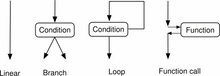Figure 1.2: Program flow

Python uses a special syntax to mark blocks of statements: a keyword, a colon, and an indented sequence of statements, which belong to the block (refer to the following Figure 1.3 Block command).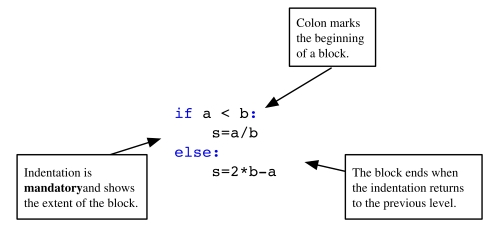Figure 1.3: Block command

If a line in a program contains the symbol `#`, everything following on the same line is considered as a comment:

```# This is a comment of the following statement
a = 3  # ... which might get a further comment here  ```

### Line joining

A backslash `\` at the end of the line marks the next line as a continuation line, that is, explicit line joining. If the line ends before all the parentheses are closed, the following line will automatically be recognized as a continuation line, that is, implicit line joining.

## Basic types

Let's go over the basic data types that you will encounter in Python.

### Numbers

A number may be an integer, a real number, or a complex number. The usual operations are:

• addition and subtraction, `+` and `-`
• multiplication and division, `*` and `/`
• power, `**`

Here is an example:

```2 ** (2 + 2) # 16
1j ** 2 # -1
1. + 3.0j```

### Note

The symbol for complex numbers

`j`  is a symbol to denote the imaginary part of a complex number. It is a syntactic element and should not be confused with multiplication by a variable. More on complex numbers can be found in section Numeric Types of Chapter 2, Variables and Basic Types.

### Strings

Strings are sequences of characters, enclosed by simple or double quotes:

```'valid string'
"string with double quotes"
'these are double quotes: ".." '```

You can also use triple quotes for strings that have multiple lines:

```"""This is
a long,
long string"""```

### Variables

A variable is a reference to an object. An object may have several references. One uses the assignment operator `=` to assign a value to a variable:

```x = [3, 4] # a list object is created
y = x # this object now has two labels: x and y
del x # we delete one of the labels
del y # both labels are removed: the object is deleted```

The value of a variable can be displayed by the `print` function:

```x = [3, 4] # a list object is created
print(x)```

### Lists

Lists are a very useful construction and one of the basic types in Python. A Python list is an ordered list of objects enclosed by square brackets. One can access the elements of a list using zero-based indexes inside square brackets:

```L1 = [5, 6]
L1 # 5
L1 # 6
L1 # raises IndexError
L2 = ['a', 1, [3, 4]]
L2 # 'a'
L2 # 3
L2[-1] # last element: [3,4]
L2[-2] # second to last: 1```

Indexing of the elements starts at zero. One can put objects of any type inside a list, even other lists. Some basic list functions are as follows:

• `list(range(n))}` creates a list with `n` elements, starting with zero:
`      print(list(range(5))) # returns [0, 1, 2, 3, 4]`
• `len` gives the length of a list:
`      len(['a', 1, 2, 34]) # returns 4`
• `append` is used to append an element to a list:
```      L = ['a', 'b', 'c']
L[-1] # 'c'
L.append('d')
L # L is now ['a', 'b', 'c', 'd']
L[-1] # 'd'```

### Operations on lists

• The operator `+` concatenates two lists:

```      L1 = [1, 2]
L2 = [3, 4]
L = L1 + L2 # [1, 2, 3, 4]```
• As one might expect, multiplying a list with an integer concatenates the list with itself several times:

`n*L` is equivalent to making n additions.

```      L = [1, 2]
3 * L # [1, 2, 1, 2, 1, 2]```

### Boolean expressions

A Boolean expression is an expression that may have the value `True` or `False`. Some common operators that yield conditional expressions are as follow:

•  Equal, `==`
•  Not equal, `!=`
•  Less than, Less than or equal to, `<` , `<=`
•  Greater than, Greater than or equal to, `>` , `>=`

One combines different Boolean values with `or` and `and`. The keyword `not` , gives the logical negation of the expression that follows. Comparisons can be chained so that, for example, `x < y < z` is equivalent to `x < y and y < z`. The difference is that `y` is only evaluated once in the first example. In both cases, `z` is not evaluated at all when the first condition, `x < y`, evaluates to `False`:

```2 >= 4  # False
2 < 3 < 4 # True
2 < 3 and 3 < 2 # False
2 != 3 < 4 or False # True
2 <= 2 and 2 >= 2 # True
not 2 == 3 # True
not False or True and False # True!```

### Note

Precedence rules

The `<`, `>`, `<=`, `>=`, `!=`, and `==` operators have higher precedence than `not.`  The operators `and`, `or` have the lowest precedence. Operators with higher precedence rules are evaluated before those with lower.

## Repeating statements with loops

Loops are used to repetitively execute a sequence of statements while changing a variable from iteration to iteration. This variable is called the index variable. It is successively assigned to the elements of a list, (refer to Chapter 9, Iterating) :

```L = [1, 2, 10]
for s in L:
print(s * 2) # output: 2 4 20```

The part to be repeated in the `for` loop has to be properly indented:

```for elt in my_list:
do_something
something_else
print("loop finished") # outside the for block```

One typical use of a `for` loop is to repeat a certain task a fixed number of times:

```n = 30
for iteration in range(n):
do_something # this gets executed n times```

### Break and else

The `for` statement has two important keywords: `break` and `else`. `break` quits the `for` loop even if the list we are iterating is not exhausted:

```for x in x_values:
if x > threshold:
break
print(x)```

The finalizing `else` checks whether the `for` loop was `broken` with the `break` keyword. If it was not broken, the block following the `else` keyword is executed:

```for x in x_values:
if x > threshold:
break
else:
print("all the x are below the threshold")```

## Conditional statements

This section covers how to use conditions for branching, breaking, or otherwise controlling your code. A conditional statement delimits a block that will be executed if the condition is true. An optional block, started with the keyword `else`  will be executed if the condition is not fulfilled (refer to Figure 1.3, Block command diagram). We demonstrate this by printing `|x|`, the absolute value of x: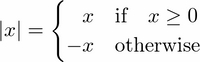The Python equivalent is as follows:

```x = ...
if x >= 0:
print(x)
else:
print(-x)```

Any object can be tested for the truth value, for use in an `if` or `while` statement. The rules for how the truth values are obtained are explained in section Boolean of Chapter 2, Variables and Basic Types.

## Encapsulating code with functions

Functions are useful for gathering similar pieces of code in one place. Consider the following mathematical function: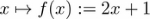The Python equivalent is as follows:

```def f(x):
return 2*x + 1```

In Figure 1.4 Anatomy of a function the  elements of a function block are explained.

• The keyword `def`   tells Python we are defining a function.
• `f` is the name of the function.
• `x` is the argument, or input of the function.
• What is after `return` is called the output of the function.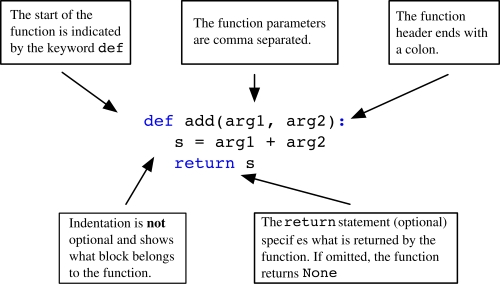Figure 1.4: Anatomy of a function

Once the function is defined, it can be called using the following code:

```f(2) # 5
f(1) # 3```

## Scripts and modules

A collection of statements in a file (which usually has a `py` extension), is called a script. Suppose we put the contents of the following code into a file named `smartscript.py`:

```def f(x):
return 2*x + 1
z = []
for x in range(10):
if f(x) > pi:
z.append(x)
else:
z.append(-1)
print(z)```

In a Python or IPython shell, such a script can then be executed with the `exec` command after opening and reading the file. Written as a one-liner it reads:

`exec(open('smartscript.py').read())`

The IPython shell provides the magic command `%run` as a handy alternative way to execute a script:

```%run smartscript
```

### Simple modules - collecting functions

Often one collects functions in a script. This creates a module with additional Python functionality. To demonstrate this, we create a module by collecting functions in a single file, for example `smartfunctions.py`:

```def f(x):
return 2*x + 1
def g(x):
return x**2 + 4*x - 5
def h(x):
return 1/f(x)```
• These functions can now be used by any external script or directly in the IPython environment.
• Functions within the module can depend on each other.
• Grouping functions with a common theme or purpose gives modules that can be shared and used by others.

Again, the command `exec(open('smartfunctions.py').read())` makes these functions available to your IPython shell (note that there is also the IPython magic function `run`). In Python terminology, one says that they are put into the actual namespace.

### Using modules and namespaces

Alternatively, the modules  can be imported by the command `import`. It creates a named namespace. The command `from` puts the functions into the general namespace:

```import smartfunctions
print(smartfunctions.f(2))      # 5

from smartfunctions import g    #import just this function
print(g(1)) # 0

from smartfunctions import *    #import all
print(h(2)*f(2))                # 1.0```

### Tip

Import

The commands `import` and `from`  import the functions only once into the respective namespace. Changing the functions after the import has no effect for the current Python session. More on modules can be found in section Modules of Chapter 11, Namespaces, Scopes and Modules.

## Interpreter

The Python interpreter executes the following steps:

• First, run the syntax.
• Then execute the code line by line.
• Code inside a function or class declaration is not executed (but checked for syntax).
```      def f(x):
return y**2
a = 3   # here both a and f are defined```

You can run the preceding program because there are no syntactical errors. You get an error only when you call the function `f`.

`f(2) # error, y is not defined`

## Summary

In this chapter, we briefly addressed the main language elements of Python without going into detail. You should now be able to start playing with small pieces of code and to test different program constructs. All this is intended as an appetizer for the following chapters in which we will give you the details, examples, exercises, and more background information.

• ##### Claus Führer

Claus Führer is a professor of scientific computations at Lund University, Sweden. He has an extensive teaching record that includes intensive programming courses in numerical analysis and engineering mathematics across various levels in many different countries and teaching environments. Claus also develops numerical software in research collaboration with industry and received Lund University’s Faculty of Engineering Best Teacher Award in 2016.

Browse publications by this author
• ##### Jan Erik Solem

Jan Erik Solem is a Python enthusiast, former associate professor, and currently the CEO of Mapillary, a street imagery computer vision company. He has previously worked as a face recognition expert, founder and CTO of Polar Rose, and computer vision team leader at Apple. Jan is a World Economic Forum technology pioneer and won the Best Nordic Thesis Award 2005-2006 for his dissertation on image analysis and pattern recognition. He is also the author of "Programming Computer Vision with Python" (O'Reilly 2012).

Browse publications by this author
• ##### Olivier Verdier

Olivier Verdier began using Python for scientific computing back in 2007 and received a PhD in mathematics from Lund University in 2009. He has held post-doctoral positions in Cologne, Trondheim, Bergen, and Umeå and is now an associate professor of mathematics at Bergen University College, Norway.

Browse publications by this author

### Latest Reviews

(3 reviews total)